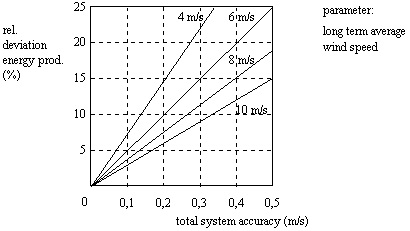ACURACY OF WIND SPEED DATA:
a key-factor in the economic analysis of wind energy projects

by PETER H. van EMDEN

#### 1. Introduction

Before an investment in wind turbines takes place a feasibility study will be carried out, which gives information to potential investors about costs and benefits (energy production) of a planned wind energy project. The Return On Investment (ROI) has been reached when the total profits are equal to the investment. The period in which the Return On Investment has been reached is a very important figure for investors, so it should be determined accurately. In this article it is clarified that the accuracy of wind speed data is a very important key-factor for the accurate determination of the period for Return On Investment.

Accurate wind speed data can save a lot of money, as wrong decisions about investment in wind turbines can be avoided. Only accurate wind speed data (accuracy: 0.1 - 0.2 m/s) can be used for this purpose. Many suppliers of wind measuring equipment do not supply equipment with the required accuracy. A scientific calibration procedure is necessary to obtain the required accuracy of the wind speed.

#### 2. The structure of a wind measuring system

Anemometer - signal conditioning and sampling - pre-processing - data collection - data processing - output
The anemometer converts the wind speed into a physical signal, usual an electrical pulse signal.
The signal conditioning converts the signal from the anemometer into an appropriate signal for sampling.
During the pre-processing the average, maximum and standard deviation of the wind speed are calculated.
During the data collection all the recorded data is stored in a memory, with sufficient resolution (at least 10 bits).
Then the data processing can take place using a PC and software which gives the results of the measurements.

#### 3. The accuracy of a wind measuring system

All system parts are contributing to the total system accuracy! The most critical system parts are the calibration of the anemometer and the way of signal conditioning and sampling.

#### 3.1 The anemometer calibration

The calibration of an anemometer takes place in a wind tunnel. As there is an interaction between anemometer and wind tunnel an accurate anemometer calibration is not just putting an anemometer in a wind tunnel: this can easily cause errors of about 0.5 m/s or more. Only a scientific calibration procedure, in which the interaction between wind tunnel and anemometer is known, can result in a calibration accuracy of up to 0.1 m/s (if carried out in a very accurate windtunnel). An individual calibration certificate is preferable, not only before a monitoring period but it is also recommended to check the calibration after this period.

For accurate measurements it is required that the complete calibration curve is taken into account. There are suppliers of wind monitoring equipment who simplify the calibration curve to a "one point calibration", or in other words: a straight line approximation, which will result in unacceptable calibration errors. For example: for the well-known MAX40 anemometer this simple approximation will result in calibration errors up to 0.5 m/s!

#### 3.2 The signal conditioning and sampling

The signal conditioning and sampling technique of the wind speed is also very important for the accuracy. When the pulses from a MAX40 anemometer are counted during a 1 second interval, the resolution of the sample values will be up to appr. 0.8 m/s! When the signal conditioning and sampling are carried out correctly (by using a special technique), the resolution of the sample values can be reduced to 0.05 m/s and for the average values 0.01 m/s.

#### 3.3 The total system accuracy

The best total system accuracy that can be realized with "low-cost" equipment is 0.1 m/s. This can be achieved by an excellent (scientific) anemometer calibration and the right way of signal conditioning and sampling.

#### 4. The accuracy of the calculated energy production

As the energy production of a wind turbine is proportional to the cube of the long-term average wind speed (v3), a small deviation in wind speed will highly affect the calculation of the energy production. The relative deviation of the calculated energy production is equal to: 3*total system accuracy / average wind speed.For example: when the yearly average wind speed is 6 m/s, measured with a system accuracy of +/- 0.5 m/s, the error in the calculation of the annual energy production is appr. +/- 25%.

#### 5. The accuracy of the period for return on investment

The period for Return On Investment (ROI) can be calculated by: ROI (years)=INVESTMENT/PROFIT (yearly average) The profit is proportional to the energy production. So the relative deviation of the calculated ROI is equal to the relative deviation of the energy production! The following examples will clarify the significant influence of the accuracy of wind speed data (refer also to the graph in section 4). In the examples the accuracy of the ROI is determined for some different cases. The values for the calculated ROI are assumed values; in reality these figures can be different! The costs for maintenance etc. are ignored. The difference between the low and high ROI strongly depends on the accuracy of the wind speed !

 Total accuracy wind speed (m/s) Yearly average wind speed (m/s) Rel. deviation of annual energy prod (+/- %) when ROI is: (Years) Accuracy of ROI (Years) 0.5 6 25 12 9 - 15 0.2 6 10 12 10.8 - 13.2 0.1 6 5 12 11.4 - 12.6 0.5 8 18.7 8 6.5 - 9.5 0.2 8 7.5 8 7.4 - 8.6 0.1 8 3.7 8 7.7 - 8.3 0.5 10 15 6 4.1 - 5.9 0.2 10 6 6 5.6 - 6.4 0.1 10 3 6 5.8 - 6.2